# Samacheer Kalvi Books: Tamilnadu State Board Text Books Solutions

## Samacheer Kalvi 7th Science Chapter 1 Heat and Temperature Notes PDF Download: Tamil Nadu STD 7th Science Chapter 1 Heat and Temperature NotesSamacheer Kalvi 7th Science Chapter 1 Heat and Temperature Notes PDF Download: Tamil Nadu STD 7th Science Chapter 1 Heat and Temperature Notes

## Samacheer Kalvi 7th Science Chapter 1 Heat and Temperature Notes PDF Download

We bring to you specially curated Samacheer Kalvi 7th Science Chapter 1 Heat and Temperature Notes PDF which have been prepared by our subject experts after carefully following the trend of the exam in the last few years. The notes will not only serve for revision purposes, but also will have several cuts and easy methods to go about a difficult problem.

 Board Tamilnadu Board Study Material Notes Class Samacheer Kalvi 7th Science Subject 7th Science Chapter Chapter 1 Heat and Temperature Format PDF Provider Samacheer Kalvi Books

## How to Download Samacheer Kalvi 7th Science Chapter 1 Heat and Temperature Notes PDFs?

2. Click on the Samacheer Kalvi 7th Science Notes PDF.
3. Look for your preferred subject.
4. Now download the Samacheer Kalvi 7th Science Chapter 1 Heat and Temperature notes PDF.

## Tamilnadu Samacheer Kalvi 7th Science Solutions Term 2 Chapter 1 Heat and Temperature

### Samacheer Kalvi 7th Science Heat and Temperature Textual Evaluation

Heat And Temperature Class 7 Samacheer Question 1.
International unit of measuring temperature is
(a) Kelvin
(b) Fahrenheit
(c) Celsius
(d) Joule
(a) Kelvin

Heat And Temperature 7th Standard Question 2.
In thermometer when bulb comes in contact with hot object, liquid inside it
(a) expands
(b) contracts
(c) remains same
(d) none of above
(a) expands

Heat And Temperature 7th Class Question 3.
The body temperature of a healthy man is;
(a) 0°C
(b) 37°C
(c) 98°C
(d) 100°C
(b) 37°C

Samacheer Kalvi Guru 7th Science Question 4.
Mercury is often used in laboratory thermometers because it
(a) is a harmless liquid
(b) is silvery in colour and is attractive in appearance
(c) Expands uniformly
(d) is a low cost liquid
(c) Expands uniformly

7th Science Heat And Temperature Question 5.
Which of the following temperature conversions is incorrect K (Kelvin) = oc ( Celsius) + 273.15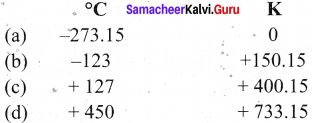II. Fill In the blanks:

1. Doctor uses ________ thermometer to measure the human body temperature.
2. At room temperature mercury is in _______ state.
3. Heat energy transfer from _______ to _______
4. -7°C temperature is _______ than 0°C temperature.
5. The common laboratory thermometer is a _______ thermometer.

1. clinical
2. liquid
3. hotter object, colder object
4. less
5. mercury

III. Match the following:

1. Clinical thermometer – A form of energy
2. Normal temperature of human body – 100°C
3. Heat – 37°C
4. Boiling point of water – o°c
5. Melting point of water – Kink

1. Clinical thermometer – Kink
2. Normal temperature of human body – 37°C
3. Heat – A form of energy
4. Boiling point of water – 100°C
5. Melting point of water – 0°C

7th Standard Science Heat And Temperature Question 1.
Temperature of Srinagar (J&K) is -4°C and in Kodaikanalis 3°C which of them
has greater temperature ? What is the difference between the temperatures of
these two places?
Kodaikanal has greater temperature.
Temperature of srinagar (J &K) = -4°C
Temperature of = 3°C
Difference = -4°C + 3°C = 7°C
Srinagar is colder than that of kodaikanal.

7th Standard Heat And Temperature Question 2.
Jyothi was prepared to measure the temperature of hot water with a clinical thermometer. Is it right or wrong? Why?
It is wrong, because clinical thermometer has small temperature range (35°C to 42°C or 94°F to 108°F). If it is used to measure the temperature of hot water, the glass will crack/ burst due to excessive pressure created by expansion of mercury.

Science 7 Heat And Temperature Question 3.
A clinical thermometer is not used to measure the temperature of air, why?
The range of the clinical thermometer is less than that of thermometer used to measure temperature of air.

Samacheer Kalvi 7th Science Term 2 Question 4.
What is the use of kink in clinical thermometer?
A kink is clinical thermometer prevents the mercury from flowing back into the bulb when the thermometer is taken out of the patient’s mouth, so that the temperature can be noted conveniently.

Samacheer Kalvi Guru 7th Science Book Back Answers Question 5.
Why do we jerk a clinical thermometer before we measure the body temperature?
The jerk to the thermometer will allow the mercury level to flow into the bulb so that the mercury level is below the normal temperature.

Heat And Temperature Class 7 Questions And Answers Question 1.
Why do we use mercury in thermometers? Can water be used instead of mercury? What are the problems in using it?

1. We use mercury in thermometers as they remain in liquid form even with a change of temperature in it.
2. A small change in the temperature causes change in volume of a liquid.
3. Water cannot be used as a thermometric liquid, because it is not helpful to measure below 0°C and above 100°C.
4. Water is transparent. So it makes the reading of the scales of the thermometer more difficult, water wets the glass tube so its steady is glass tube.
5. Due to this constraints it is not used as a thermometric liquid.

Heat And Temperature Answer Key Question 2.
Swathi kept a laboratory thermometer in hot water for some time and took it out to read the temperature. Ramani said it was a wrong way of measuring temperature. Do you agree with Ramani ? Explain your answer.
Yes, I agree with Ramani.

1. Laboratory thermometer does not have a kink. So, when Swathi takes out the thermometer, the level can go back because of absence of kink.
2. So Swathi should note the reading when the thermometer bulb has surrounded by hot water.

Question 3.
The body temperature of Srinath is 99°F. Is he suffering from fever? If so, why?
Srinath is having a fever because the normal body temperature is 98.6°F.

Question 1.
Draw the diagram of a clinical thermometer and label its parts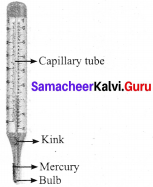Question 2.
State the similarities and differences between the laboratory thermometer and the clinical thermometer.
Similarities between laboratory thermometer and the clinical thermometer:

1. Both clinical and laboratory thermometers have long, narrow and uniform glass tubes.
2. Bulbs contain mercury.
3. Both have Celsius scale.

Differences:

Laboratory thermometer:

1. Laboratory thermometer is generally scaled from -10°C to 11 CPC.
2. Mercury level falls on its own as no kink is present.
3. Temperature is read while keeping the thermometer in the source of temperature, e.g. a liquid or any other thing.
4. No need to give jerk to lower the mercury level.
5. It is used to take temperature in laboratory.

Clinical thermometer:

1. Clinical thermometer is scaled from 35°C to 42°C or from 94°F to 108°F.
2. Mercury level does not fall on its own, as there is a kink near the bulb to prevent the fall of mercury level.
3. Temperature can be read after removing the thermometer from armpit or mouth.
4. To lower the mercury level jerks are given.
5. It is used for taking the body temperature.

VII. Higher Order Thinking questions:

Question 1.
What must be the temperature in Fahrenheit, so that it will be twice its value in Celsius?
According to the question, F = 2C and C1 = C
F = 9C5 + 32
2c =95 C + 32
2c – 95 c = 32 ⇒10c9c5 = 32 + 0
1c5 = 32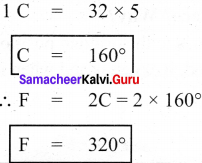Question 2.
Go to a veterinary doctor (a doctor who treats animals). Discuss and find out the normal temperature of domestic animals and birds.

1. Dog – 38.9°C
2. Horse – 38°C
3. Rabbit – 38.3°C
4. Cow – 38.6°C
5. Cat – 39°C
6. Goat- 39.7°C
7. Pigeon – 44.1°C
8. Crow – 40°C
9. Duck – 40.7°C
10. Karaknath – 41.8°C
11. Parrot – 41°C

### Samacheer Kalvi 7th Science Heat and Temperature Intext Activites

Activity – 1

Question 1.
What is required?
A small glass bottle, a rubber cork, an empty refill, water, colour, a candle, a fork, a paper.
What to do?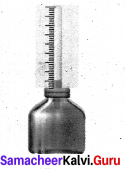• Take a small glass bottle. Fill it with coloured water.
• Make hole at the centre of the rubber cork.
• Pass empty refill from the hole of the rubber cork.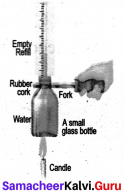• Make the bottle air tight and observe the water raised in the refill.
• Make a scale on paper, place it behind the refill and note down the position of the surface of water.
• Hold bottle with fork and supply heat to it with candle. Then ‘ observe.

Question 2.
What is the change in the surface of water?
The level of water increases.
Stop the supply of heat. When water is cooled, observe the surface of water in the refill,

Question 3.
what change takes place? Why?
The level of water comes to the original position.
When, a liquid is heated, it expands and when it is cooled down, it contracts.

Activity – 2

Question 1.
What is required?
A big bottle, a balloon, threads, candle, water, fork.
What to do?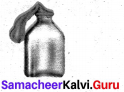• Take one big bottle, and fill some water in it.
• Attach one balloon on the mouth of bottle and fix it with thread.
• Hold bottle with a fork. Heat the bottle with a candle and take observation.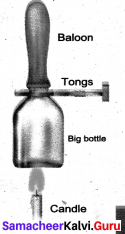Question 2.
What change occurs in the state of balloon after heating the bottle?
The balloon expands.

Question 3.
What change occurs in the state of balloon after heating the bottle?
Why?
The air inside it gets heated and expands. This causes the balloon to stretch and expand.
Now, let the bottle get cooled down.

Question 6.
What change occurs in the state of balloon after bottle gets cool down?
Why?
The temperature within the bottle reduces causing the air to cool down. This causes the balloon to contract.
When gases substance gets heat, it expands; when it cools it contracts.

Question 5.
Why does a tyre get burst in summer?
During summer, the air inside the tyre of a vehicle will get heated up with the increase in pressure of the air inside the tyre. As there is no place to air to escape tyre will get bursted.

Activity – 3
Wash the thermometer preferably with an antiseptic solution. Hold it firmly by the end and give it a few jerks. These jerks will bring the level of Mercury down. Ensure that it falls below 35°C (95°F). Now place the thermometer under your tongue or arm pit. After one minute, take the thermometer out and note the reading. It tells you your body temperature.
What did you record as your body temperature?
37°C

1.6 Numerical problems:

3. Convert the given temperature :

Question 1.
45°C = _____ °F
Solution:
F = 9c5 + 32
9×4598
= 81 +32
45°c = 113°F

Question 2.
20°C =______°F
Solution:
F =9c5
9×2045 + 32
=36 + 32
20°c = 68°F

Question 3.
68°F =_______°F
Solution:
C = (F-32) ×59
= (68 – 32) × 59
= 36 ×59
68°F = 20°C

Question 4.
185°C =_______°F
Solution:
c = (F – 32) × 59
= (185 -32) × 59
= 153 × 59
185F° = 85°C

Question 5.
0°=________°F
Solution:
K = C + 273
= 0 + 273
0°C = 273K

Question 6.
– 20°=________°F
Solution:
k = C + 273
= – 20 + 273
– 20°C = 273K

Question 7.
100K=________°F
Solution:
C = K – 273
=272.15 – 273
272.15K= – 0.85°C

### Samacheer Kalvi 7th Science Heat and Temperature Additional Questions

Question 1.
Heat energy is the total of the particles that make up a substance.
(a) potential energy
(b) substance
(c) temperature
(d) none
(b) substance

Question 2.
Heat energy is always transferred from to temperatures.
(a) lower, higher
(b) higher, lower
(c) conductor, insulator
(d) both a and b
(b) higher, lower

Question 3.
A clinical thermometer is calibrated from to .
(a) 10°Ctol00°C
(b) 32°C to 110°C
(c) 0°Ctol00°C
(d) 35°C to 42°C
(d) 35°C to 42°C

Question 4.
The thermometer which ranger from -10°C to 110°C is .
(a) clinical thermometer
(b) digital thermometer
(c) laboratory thermometer
(d) All of these
(c) laboratory thermometer

Question 5.
Which one of the following scale has lower fixed point at 0°C?
(a) Kelvin scale
(b) Fahrenheit scale
(c) Celsius scale
(d) All of these
(c) Celsius scale

Question 6.
The lower fixed point on the Celsius scale is
(a) melting point of mercury
(b) melting point of ice
(c) boiling point of water
(d) none
(b) melting point of ice

Question 7.
The measure of degree of hotness or coldness of a body is called
(a) heat energy
(b) Celsius
(c) kelvin
(d) temperature
(d) temperature

Question 8.
Digital thermometer is widely used for the measurement of the temperature due to .
(a) Analog display
(b) Mercury
(c) High accuracy
(d) Low accuracy
(c) High accuracy

Question 9.
Temperature of boiling water cannot be measured by a thermometer.
(a) laboratory
(b) digital
(c) clinical
(d) both b & c
(d) both b & c

Question 10.
When a substance is heated, its temperature .
(a) rises
(b) falls
(c) remains same
(d) none
(a) rises

II. Fill in the Blanks.

1. Digital thermometers do not use ______
2. In a thermometer, when liquid gets heat, it_____and when it is cooled down, it
3. The SI unit of temperature is ______
4. A small change in the temperature causes change in of a liquid.________
5. The freezing point of alcohol is less than________
6. All clinical thermometers have a________ that prevents mercury from flowing back into the bulb.
7. A clinical thermometer indicates temperatures from a minimum of ________ °F to a maximum of °F.
8. Before use, the mercury level in clinical thermometer should be below________
9. The coldest natural temperature ever recorded on earth is________
10. The hottest natural temperature ever recorded on earth is________
11. Temperature of the universe in the earliest moments after the big bang is ________

1. mercury
2. expand, contact
3. kelvin
4. volume
5. – 100°
6. kink
7. 94° F,108° F
8. 35° C or 94° F
9. 178.45K
10. 392.85K
11. 10³² K

III. True or False – if false give the correct statement.

Question 1.
Temperature is related to how fast the atoms within a substance are moving.
True

Question 2.
Mercury is a poor conductor of heat.
False. Mercury is a good conductor of heat.

Question 3.
Fahrenheit scale is more sensitive that the Celsius scale.
True

Question 4.
In humans, the average internal temperature is 98.60°C.
False. In humans, the average internal temperature is 98.60°F.

Question 5.
Digital thermometers are mainly used to take the body temperature.
True

Question 6.
The glass of a clinical thermometer will burst due to excessive pressure created by contraction of mercury.
The glass of a clinical thermometer will burst due to excessive pressure created by expansion of mercury.

Question 7.
In greek, Centium means 100 and Gradus means steps, both words make it Centigrade and later Celsius.
True

Question 8.
Kelvin is written as K also known as absolute scale as it starts from absolute zero temperature.
True

Question 9.
The temperatures in Celsius and in Fahrenheit will be same at -40.
True

IV. Match the following :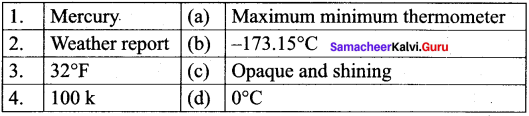1. (c)
2. (a)
3. (d)
4. (b)

V. Assertion and Reason.
Mark the correct choice as
(a) Both A and R are true but R is the correct explanation of assertion.
(b) Both A and R are true but R is not correct explanation of assertion.
(c) A is true but R is false.
(d) A is false but R is true.

1. Assertion

(A): Heat energy is transferred from one body to another due to a temperature difference between them.
Reason (R): Heating a substance causes a rise in temperature.
(b) Both A and R are true but R is not correct explanation of assertion.

Correct reason: Heat energy always travels from hotter object to colder objects.

1. Assertion

(A): When a very hot liquid is poured into a thick glass tumbler it cracks.
Reason (R): Unequal expansion of inner and outer glass walls causes the glass to crack.
(a) Both A and R are true but R is the correct explanation of assertion

Question 1.
Mention the three units which are used to measure the temperature.
The three units which are used to measure the temperature: Degree Celsius, Fahrenheit and Kelvin.

Question 2.
What is the boiling point of mercury?
357°C

Question 3.
What is the freezing point of alcohol?
Less than -100°C

Question 4.
Mention the use of laboratory thermometers.
To measure the temperature is laboratories for scientific research.

Question 5.
What is the average internal temperature of human body?
37°C (98.6°F)

Question 6.
What is the freezing point of water is Fahrenheit scale?
32°F

Question 7.
At what temperature the value will be same in Celsius and in Fahrenheit?
– 40.

Question 8.
Name the principle which is used in thermometer.
Liquids expand on heating and contract on cooling.

Question 1.
Mention any two precautions to be followed while using a clinical thermometer.

1. The thermometer should be washed before and after use, preferably with an antiseptic solution.
2. Jerk the thermometer a few times to bring the level of the mercury down.

Question 2.
What are the uses of laboratory thermometers?
Laboratory thermometers are used to measure the temperature in school and other laboratories for scientific research. They are also used in the industry as they can measure temperatures higher than what clinical thermometers can record.

Question 3.
Mention any two properties of alcohol.

1. The freezing point of alcohol is less than -100°C. So it can be used to measure very low temperatures.
2. Its expansion per degree Celsius rise in temperature is very large.

Question 4.
What is the use of kink in clinical thermometers?
Kink prevents the mercury from flowing back into the bulb when the thermometer is taken out of the patient’s mouth. So that the temperature can be noted conveniently.

Question 5.
Why mercury or alcohol is used in thermometers?
Mostly Alcohol and Mercury are used in thermometers as they remain in liquid form even with a change of temperature in them. A small change in the temperature causes change in volume of a liquid. We measure this temperature by measuring expansion of a liquid in thermometer.

Question 6.
We are advised to avoid keeping clinical thermometer in the sun or near a flame. Why?
A Clinical thermometer has small temperature range. The glass will crack/ burst due to excessive pressure created by expansion of mercury.

Question 1.
Explains the properties of mercury.

1. Its expansion is uniform. (For equal amounts of heat it expands by equal lengths.)
2. If is opaque and shining.
3. It does not stick to the sides of the glass tube.
4. It is a good conductor of heat.
5. It has a high boiling point (357°C) and a low freezing point (-39°C). Hence a wide range of temperatures can be measured using a mercury thermometer.

Question 2.
Explain the construction and working of clinical thermometer.

1. Clinical thermometers are used to measure the temperature of a human body – at home, clinics and hospitals.
2. All clinical thermometers have a kink that prevents the mercury from flowing back into the bulb when the thermometer is taken out of the patient’s mouth, so that the temperature can be noted conveniently.
3. There are temperature scales on either side of the mercury thread, one in C elsius scale and the other in Fahrenheit scale.
4. Since the Fahrenheit scale is more sensitive than the Celsius scale, body temperature is measured in F only.
5. A clinical thermometer indicates temperatures from a minimum of 35°C or 94°F to a maximum of 42°C or 108°F.

Question 3.
Explain the precautions to be followed while using clinical thermometer.

1. The thermometer should be washed before and after use, preferably with an antiseptic solution.
2. Jerk the thermometer a few times to bring the level of the mercury down.
3. Before use, the mercury level should be below 35°C or 94°F.
4. Do not hold the thermometer by its bulb.
5. Keep the mercury level along your line of sight and then take the reading.
6. Handle the thermometer with care. If it hits against some hard object, it may break.
7. Do not place the thermometer in a hot flame or in the hot sun.

Question 4.

1. Write a note on laboratory thermometer.
2. Write the precautions to be following while using a laboratory thermometer.

(a) Laboratory Thermometers:

1. Laboratory thermometers are used to measure the temperature in school and other laboratories for scientific research.
2. They are also used in the industry as they can measure temperatures higher than what clinical thermometers can record.
3. The stem and the bulb of a lab thermometer are longer when compared to that of a clinical thermometer and there is no kink in the lab thermometer.
4. A laboratory thermometer has only the Celsius scale ranging from -10°C to 110°C.

(b) Precautions to be Followed While Using a Laboratory Thermometer:

1. Do not tilt the thermometer while measuring the temperature. Place it  upright.
2. Note the reading only when the bulb has been surrounded by the substance from all sides.

Question 5.
Explain how do you determine the temperature of hot water using laboratory thermometer?

1. Take some water in a beaker.
2. Take a laboratory thermometer and immerse its bulb end in water; holding it vertically. Ensure to dip whole portion of bulb end. The bulb end should not touch the bottom or side of the beaker.
3. Observe the movement of rise of mercury. When it becomes stable, take the reading of the thermometer.
4. Repeat this with hot water and take the reading.

Question 6.

1. write a note on digital thermometer.
2. Mention the precaution to be followed while using digital thermometer.

(a) Digital Thermometer:

1. Mercury is a toxic substance and is very difficult to dispose of if a thermometer breaks.
2. These days, digital thermometers are available which do not use mercury.
3. Instead, it has a sensor which can measure the heat coming out from the body directly and from that can measure the temperature of the body.
4. Digital thermometers are mainly used to take the body temperature.
5. Do not use a clinical thermometer for measuring the temperature of any object other than human body.
6. Avoid keeping it in the sun or near of flame.

Question 7.
Explain the usage of digital thermometer.
Use of Digital thermometer:

1. Wash the tip with warm (not hot), soapy water.
2. Press the “ON” button.
3. Insert the tip of the thermometer into the mouth, bottom, or under the armpit.
4. Hold the thermometer in place until it beeps (about 30 seconds).
6. Turn off the thermometer, rinse under water, and put it away in a safe place.

IX.Problems for practice:
Convert the given temperature:

Question 1.
40°C =_______°F
Solution:
F = 9c5 +32
9×4088 + 32
= 72 + 32
40°C = 104°F

Question 2.
0 K =_______°F
Solution:
C = k – 273
= 0 – 273
0 K = – 273°C

Question 3.
– 30°C = _______°F
Solution:
K = C + 273
= – 30 + 273

Question 4.
150°F =_______°C
Solution:
C = (F – 32) × 59
= 118 × 59
=5909 = 65.9
150°F = 65.9°C

X . Creative Questions: HOTS

Question 1.
What the minimum possible temperature is 0 k .There also a maximum possible temperature ?
The minimum possible temperature is 0 K. There is no limit to maximum temperature.

Question 2.
Complete the table.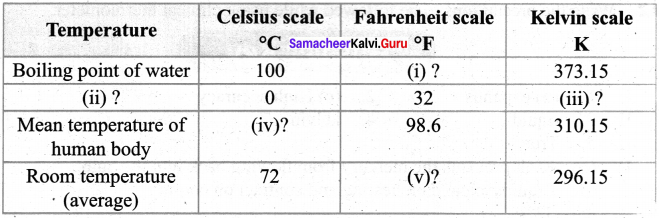1. 212°F
2. Freezing point of water
3. 273.15 K
4. 37°C
5. 23 K

## How to Prepare using Samacheer Kalvi 7th Science Chapter 1 Heat and Temperature Notes PDF?

Students must prepare for the upcoming exams from Samacheer Kalvi 7th Science Chapter 1 Heat and Temperature Notes PDF by following certain essential steps which are provided below.

• Use Samacheer Kalvi 7th Science Chapter 1 Heat and Temperature notes by paying attention to facts and ideas.
• Pay attention to the important topics
• Refer TN Board books as well as the books recommended.
• Correctly follow the notes to reduce the number of questions being answered in the exam incorrectly
• Highlight and explain the concepts in details.

## Frequently Asked Questions on Samacheer Kalvi 7th Science Chapter 1 Heat and Temperature Notes

#### How to use Samacheer Kalvi 7th Science Chapter 1 Heat and Temperature Notes for preparation??

Read TN Board thoroughly, make separate notes for points you forget, formulae, reactions, diagrams. Highlight important points in the book itself and make use of the space provided in the margin to jot down other important points on the same topic from different sources.

#### How to make notes for Samacheer Kalvi 7th Science Chapter 1 Heat and Temperature exam?

Read from hand-made notes prepared after understanding concepts, refrain from replicating from the textbook. Use highlighters for important points. Revise from these notes regularly and formulate your own tricks, shortcuts and mnemonics, mappings etc.
Share: